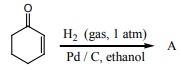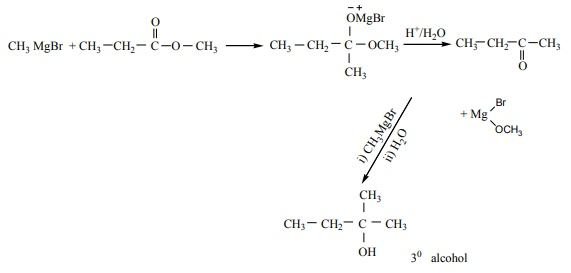# TN 12TH CHEMISTRY - Online Test

Q1. For Freundlich isotherm a graph of log (x/m) is plotted against log p. The slope of the line and its y – axis intercept respectively corresponds to
Explaination / Solution:

x/m =k.p1/n

log(x/m )=logk+ 1/ n logp

y=c+mx

m = 1/n and c= logk

Q2. An alcohol (x) gives blue colour in Victormeyer’s test and 3.7g of X when treated with metallic sodium liberates 560 mL of hydrogen at 273 K and 1 atm pressure what will be the possible structure of X?
Explaination / Solution:

2 R - OH + 2Na 2 RONa + H22 moles of alcohol gives 1 mole of H2 which occupies 22.4L at 273K and 1 atm

number of moles of alcohol = ( 2 moles of R - OH / 22.4 L of H2 ) × 560 mL

= 0.05 moles

no. of moles = mass / molar mass

molar mass = 3.7/0.05 = 74 g mol1

General formula for R - OH Cn H2n+1 - OH

n(12) + (2n+1) (1) + 16+1=74

14n = 74 – 18

14n = 56

n = 56/14 = 4

The 2 alcohol which contains 4 carbon is CH2 CH(OH)CH2CH3

Q3. The correct structure of the product ‘A’ formed in the reactionis
Explaination / Solution:
No Explaination.

Q4. Which of the following reagent can be used to convert nitrobenzene to aniline
Explaination / Solution:
No Explaination.

Q5. Which one of the following rotates the plane polarized light towards left?
Explaination / Solution:
No Explaination.

Q6. Which of the following is an analgesic?
Explaination / Solution:
No Explaination.

Q7. Concentration of the Ag+ ions in a saturated solution of Ag2 C2O4 is 2.24 ×10-4mol L-1 solubility product of Ag2 C2O4 is
Explaination / Solution:

Ag2C2O4 2Ag+ + C2O42-

[ Ag+ ] = 2.24 × 10-4 mol L-1

[ C2 O42- ] = { 2.24×10-4 }/2 = mol L-1

= 1.12×10-4 mol L-1

Ksp = [Ag+]2[C2O42-]

= (2.24×10-4 mol-4 L-1 )2 (1.12×10-4 mol L-1)

= 5.619×10-12mol3 L-3

Q8. The number of electrons that have a total charge of 9650 coulombs is
Explaination / Solution:

1F = 96500 C = 1 mole of e = 6.023 ×1023 e

9650 C = [ 6.22 ×1023 / 96500 ] × 9650 = 6.022×1022

= 6.022×1022

Q9. Which of the following is incorrect for physisorption?
Explaination / Solution:

The incorrect statement is option (b)

Physisorption is an exothermic process. Hence increase in temperature decreases the physisorption.

Q10. Which of the following compounds on reaction with methyl magnesium bromide will give tertiary alcohol.
Explaination / Solution: